Courses

# Short Answer Type Questions (Part 2): Real Numbers Class 10 Notes | EduRev

## Class 10 : Short Answer Type Questions (Part 2): Real Numbers Class 10 Notes | EduRev

The document Short Answer Type Questions (Part 2): Real Numbers Class 10 Notes | EduRev is a part of the Class 10 Course Class 10 Mathematics by VP Classes.
All you need of Class 10 at this link: Class 10

Ques 19: Prove that 5-2√3 is an irrational number.
Sol: Let 5-2√3 is a rational number
∴ 5-2√3= p/q  where p and q are co-prime integers and q ≠ 0.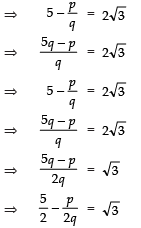Since, p and q are integers.
∴  p/2q is a rational number
i.e.,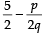is a rational number.
⇒√3 is a rational number.
But this contradicts the fact that √3 is an irrational number.
So, our assumption that (5-2√3) is a rational number is not correct.
∴ (5-2√3) is an irrational number.

Ques 20: Prove that (5+32) is an irrational number.
Sol: Let (5+3√2) is a rational number.
∴ (5+3√2) =  a/b [where ‘a’ and ‘b’ are co-prime integers and b ≠ 0]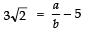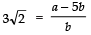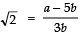‘a’ and ‘b’ are integers,
∴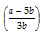is a rational number.
⇒ √2 is a rational number.
But this contradicts the fact that √2 is an irrational number.
∴ Our assumption that (5+3√2) is a rational is incorrect.
⇒ (5+3√2) is an irrational number.

Ques 21: Use Euclid’s Division Lemma to show that the square of any positive integer is either of the form ‘3m’ or ‘3m + 1’ for some integer ‘m’.
Sol: Let x be a positive integer
∴ x can be of the form 3p, (3p + 1) or (3p + 2)
When x = 3p,  we have
x2 = (3p)2
⇒ x2 = 9p2
⇒ x2  = 3 (3p2)
⇒ x2 = 3m [Here 3p2 = m]
When x  = (3p + 1),  we have
x=  (3p + 1)2
⇒ x2 = 9p2 + 6p + 1
⇒ x2 = 3p (3p + 2) + 1
= 3m + 1,
Where m =p (3p +2)
When x   = 3p +2 ,  we have
x= (3p + 2)2
= 9p2 + 12p + 4
= 9p2 + 12p + 3 + 1
= 3 (3p2 + 4p + 1) + 1
= 3m + 1,  where
m  = 3p2 + 4p + 1
Thus, x2 is of the form 3m or 3m + 1.

Ques 22: Show that 2+√3 is an irrational number.
Sol:
Let  2+√3 is a rational number.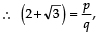such that p and q are co-prime integers and q ≠ 0.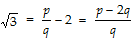Since, p and q are integers.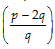is rational
⇒ √3 is rational.
But this contradicts the fact that √3  is an irrational.
∴ Our assumption that (2+√3) is a rational is incorrect.
Thus, (2+√3) is an irrational.

Ques 23: Show that one and only one of n, n + 2 and n + 4 is divisible by 3.
Sol: Let us divide n by 3.
Let us get ‘q’ as quotient and ‘r’ remainder.
∴ n  = 3 × q + r,where 0 ≤ r < 3
i.e., r  =  0, 1, 2
when r = 0,  then  n = 3q   ... (1)
when r = 1,  then  n = 3q + 1   ... (2)
when r = 2,  then  n = 3q + 2    ... (3)
From (1), n is divisible by 3
From (2), n = 3q + 1
Adding 2 to both sides, we have
n + 2 = (3q + 1) + 2
⇒ n + 2 = 3q + 3
⇒ n + 2 = 3 (q + 1)
3 (q + 1) is divisible by 3,
∴  n + 2 is divisible by 3.
From (3),
n = 3q + 2
Adding 4 to both sides,
(n + 4) = (3q + 2) + 4
⇒ n + 4 = 3q + 6 = 3 (q + 2)
3 (q + 2) is divisible by 3.
∴  n + 4 is divisible by 3.
At one time, r has only one value out of 0, 1, 2. Hence, only one of n, n + 2, n + 4 is divisible by 3.
OR
Let q be an integer such that 3q, (3q + 1) or (3q + 2) is a positive integer. Let us consider the following cases :
Case-I: When n = 3q
3q ÷ 3, gives 0 as remainder
∴  n = 3q is divisible by 3.
Next n = 3q ⇒ n + 2 = 3q + 2
(3q + 2) ÷ 3, gives 2 as remainder.
⇒ n + 2 = (3q + 2) is not divisible by 3.
Again, n = 3q ⇒  n + 4 = 3q + 4 = (3q + 3) + 1
∴ [(3q + 3) + 1] ÷ 3, gives 1 as remainder.
⇒ n + 4 = (3q + 4) is not divisible by 3.
Thus, n is divisible by 3, but (n + 2) and (n + 4) are not divisible by 3.

Case-II: When n = 3q + 1
Since (3q + 1) ÷ 3, gives remainder as 1.
∴ n = (3q + 1) is not divisible by 3.
Next, n + 2 = (3q + 1) + 2 = (3q + 3) + 0
[(3q + 3) + 0] ÷ 3, gives remainder as 0
⇒ n + 2 = (3q + 1) + 2 is divisible by 3.
Again, n = (3q + 1) ⇒  n + 4 = (3q + 1) + 4 = (3q + 3) + 2
[(3q + 3) + 2] ÷ 3, gives remainder as 2
⇒ n + 4 = (3q + 1) + 4 is not divisible by 3.
Thus, (n + 2) is divisible by 3, but n and (n + 4) are not divisible by 3.

Case-III: When n = 3q + 2
Since (3q + 2) ÷ 3, gives remainder as 2
∴ n = 3q + 2 is not divisible by 3
Next n + 2 = (3q + 2) + 2 = (3q + 3) + 1
[(3q + 3) + 1] ÷ 3, gives remainder as 1.
⇒ n + 2 = (3q + 2) + 2 is not divisible by 3
Again, n = 3q + 2   ⇒ n + 4 = (3q + 2) + 4 = (3q + 6) + 0
[(3q + 6) + 0] ÷ 3, gives remainder as 0
⇒ n + 4 = (3q + 2) + 4 is divisible by 3
Thus, (n + 4) is divisible by 3, but n and (n + 2) are not divisible by 3.

Ques 24: Show that (2+√5 )is an irrational number.
Sol:
Let (2+√5)  is a rational number.
∴ (2+√5) = p/q  , such that p and q are co-prime integers and q ≠ 0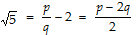p and q are integers.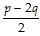is a rational.
⇒ √5 is a rational.
But, this contradicts the fact that √5 is an irrational.
∴ Our supposition that (2+√5) is rational is incorrect.
Thus,(2+√5)is an irrational.

Ques 25: Using Euclid’s division algorithm, find the HCF of 56, 96 and 404.
Sol: Using the Euclid’s division algorithm for 56 and 96
We have 96 = 56 × 1 + 40  and
56 = 40 × 1 + 16
Similarly 40 = 16 × 2 + 8
and 16 = 8 × 2 + 0
Remainder is zero
∴ HCF of 56 and 40 is 8.
Now, 404 = 8 × 50 + 4
and 8 = 4 × 2 + 0
∵ Remainder is zero
∴ HCF of 404 and 8, is 4
Thus, the HCF of 56, 96 and 404 is 4.

Ques 26: Prove that 3-√5 is an irrational number.
Sol: Let (3-√5) is a rational number.
∴ 3-√5 = p/q , such that p and q are co-prime integers and q ≠ 0.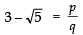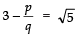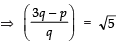Since, p and q are integers,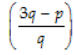is a rational number.
⇒ √5 is a rational number.
But this contradicts the fact that √5 is an irrational number.
∴ Our assumption that (3-√5)  is a rational number’ is incorrect.
⇒ (3-√5)  is an irrational number.

Ques 27: Prove that (5+ √2) is irrational.
Sol: √2 = a/b where ‘a’ and ‘b’ are co-prime integers and b ≠ 0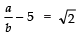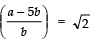since ‘a’ and ‘b’ are integers,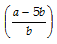is a rational.
2 is a rational.
But this contradicts the fact that 2 is an irrational.
∴ Our assumption that (5+ √2) is a rational number is incorrect.
Thus, (5+ √2) is an irrational number.

Ques 28: Prove that 23-7 is an irrational.
Sol: Let  2√3-7 is rational.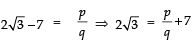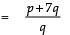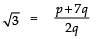∵p and q are integers.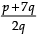is rational.
⇒ √3 is rational.
But we know that √3 is irrational,
∴ Our assumption that (2√3-7) is rational is wrong.
Hence 2√3-7 is irrational.

Ques 29: If ‘n’ in an integer, then show that n2 – 1 is divisible by 8.
Sol:
Le q be an integer, then
4q + 1 or 4q + 3
Now,
Case-I: When
n = 4q + 1
∴ n= (4q + 1)2 = 16q2 + 8q + 1
⇒ n2 – 1 = 16q2 + 8q + 1 – 1
= 16q2 + 8q
= 8q (2q + 1), which is divisible by 8.
⇒ n2 – 1 is divisible by 8.

Case-II: When
n = 4q + 3
∴ n2  =  (4q + 3)2 = 16q2 + 24q + 9
⇒ n2 – 1=  16q2 + 24q + 9 – 1 = 16q2 + 24q + 8
= 8(2q2 + 3q + 1) which is divisible by 8.
⇒ n2 – 1 is divisible by 8.
Thus, (n2 – 1) is divisible by 8.

Ques 30: Prove that if x and y are both odd positive integers, then x2 + y2 is even but not divisible by 4.
Sol: Let ‘q’ be an integer, then any odd positive integer is of the form 2q + 1
Again let for some integers m and n are such that
x = 2m + 1 and y = 2n + 1
∴ x2 + y2 = (2m + 1)2 + (2n + 1)2
= 4m2 + 4m + 1 + 4n2 +4n + 1
= 4(m2 + n2) + 4
(m + n) + 2
= 4[(m2 + n2) + (m + n)] + 2
= 4q + 2
[Where q = (m2 + n2) + (m + n)]
⇒  x2 + y= 2[2q + 1], which an even number
Thus, 4q + 2 is an even number that is not divisible by 4, i.e. it leaves remainder 2.
Hence, x2 + y2 is even but not divisible by 4.

Ques 31: Show that the square of an odd positive integer can be of the form 6q + 1 or 6q + 3 for some integer q.
Sol: Let ‘m’ be an integer such that 6m, 6m + 1, 6m + 2, 6m + 3, 6m + 4 or 6m + 5 can be any positive integer.
∴ An odd positive integer can be of the form 6m + 1, 6m + 3  or 6m + 5
Now, we have
(6m + 1) =  36m2 + 12m + 1
= 6(6m2 + 2m) + 1
= 6q + 1, q is an integer
(6m + 3)2  =  36m2 + 36m + 9
= 6(6m2 + 6m + 1) + 3
= 6q + 3, q is an integer
(6m + 5)= 36m2 + 60m + 25
= 6(6m2 + 10m + 4) + 1
= 6q + 1,  q is an integer
Thus, the square of an odd positive integer can be of the form 6q + 1 or 6q + 3.

Ques 32: Show that one and only one out of n, n + 4, n + 8, n + 12 and n + 16 is divisible by 5, where n is any positive integer.
Sol:
Let q be an integer such that 5q, (5q + 1) or (5q + 2) is a positive integer. Let us consider the following cases:
Case-I: When n = 5q
Since 5q ÷ 5, gives remainder as 0 ⇒ n is divisible by 5
Next, n = 5q ⇒ n + 4 = 5q + 4
⇒ (n + 4) is not divisible by 5
and (5q + 4) ÷ 5, gives remainder as 4
Again,  n = 5q ⇒ n + 8 = 5q + 8 = (5q + 5) + 3
⇒ (n + 8) is not divisible by 5
and [(5q + 5) + 3] ÷ 5, gives remainder as 3
Similarly,
n = 5q ⇒ n + 12 = 5q + 12 = (5q + 10) + 2
⇒ (n + 12) is not divisible by 5
and [(5q + 10) + 2] ÷ 5, gives remainder as 2
n = 5q ⇒ n + 16 = 5q + 16 = (5q + 15) + 1
⇒ (n + 16) is not divisible by 5
and [(5q + 15) + 1] ÷ 5, gives remainder as 1
Thus, n is divisible by 5, but (n + 4), (n + 8), (n + 12) and (n + 16) are not divisible by 5.

Case-II: When n = (5q + 1)
Here, (5q + 1) ÷ 5, gives remainder as 1 ⇒ n is not divisible by 5
Next  n = (5q + 1)
⇒ n + 4 = (5q + 1) + 4 = (5q + 5) + 0
⇒ (n + 4) is divisible by 5
and [(5q + 5) + 0] ÷ 5, gives remainder as 0
Similarly,
n = (5q + 1) ⇒ n + 8 = (5q + 1) + 8
= (5q + 5) + 4
⇒ (n + 8) is not divisible by 5
and [(5q + 5) + 4] ÷ 5 gives remainder as 4
n = (5q + 1) ⇒ n + 12  = (5q + 1) + 12
= (5q + 10) + 3
⇒ (n + 12) is not divisible by 5
and [(5q + 10) + 3] ÷ 5, gives remainder as 3
n = 5q + 1 ⇒ n + 16  = 5q + 1 + 16
= (5q + 15) + 2
⇒ (n + 16) is not divisible by 5
and [(5q + 15) + 2] ÷ 5, gives remainder as 2
Thus, (n + 4) is divisible by 5, but n, (n + 8), (n + 12) and (n + 16) are not divisible by 5.

Case-III: When n = (5q + 2)
Here, (5q + 2) ÷ 5, gives remainder as 2
⇒ n is not divisible by 5
Next n = 5q + 2 ⇒ n + 4 = 5q + 2 + 4
⇒ 5q + 6 = (5q + 5) + 1
⇒ (n + 4) is not divisible by 5
and [(5q + 5) + 1] ÷ 5, gives remainder as 1
Similarly,
n = 5q + 2 ⇒ n + 8 = 5q + 2 + 8 = 5q + 10
⇒ (n + 8) is divisible by 5
and (5q + 10) ÷ 5, gives remainder as 0
n = 5q + 2 ⇒ n + 12 = 5q + 2 + 12
= (5q + 10) + 4
⇒ (n + 12) is not divisible by 5
and [(5q + 10) + 4] ÷ 5, gives remainder as 4
n = 5q + 2 ⇒ n + 16 = 5q + 2 + 16
= (5q + 15) + 3
⇒ (n + 16) is not divisible by 5
and [(5q + 15) + 3] ÷ 5, gives remainder 3
Thus, (n + 8) is divisible by 5, but n, (n + 4) (n + 12) and (n + 16) are not divisible by 5.

Case-IV: When n = (5q + 3)
Here, (5q + 3) ÷ 5, gives remainder as 3 ⇒ n is not divisible by 5.
Next n = 5q + 3 ⇒ n + 4 = (5q + 3) + 4
= (5q + 5) + 2
⇒ (n + 4) is not divisible by 5
and [(5q + 5) + 2] ÷ 5, gives remainder as 2
Similarly,
n = 5q + 3 ⇒ n + 8 = 5q + 3 + 8
= (5q + 10) + 1
⇒ (n + 8) is not divisible by 5
and [(5q + 10) +1] ÷ 5, gives remainder as 1
n = 5q + 3 ⇒ n + 12 = 5q + 3 + 12
= (5q + 15) + 0
⇒ (n + 12) is divisible by 5
and [(5q + 15) + 0] ÷ 5, gives remainder as 0
n = 5q + 3 ⇒ n + 16 = 5q + 3 + 16
= (5q + 15) + 4
⇒ (n + 16) is not divisible by 5
and [(5q + 15) + 4] ÷ 5, gives remainder as 4
Thus, (n + 16) is divisible by 5 but n, (n + 4) , (n + 8) and (n + 12) are not divisible by 5.

Case-V: When n = (5q + 4)
Here, (5q + 4) ÷ 5, gives remainder as 4 ⇒ n is not divisible by 5
Next, n = (5q + 4) ⇒ n + 4 = 5q + 4 + 4
= (5q + 5) + 3
⇒ (n + 4) is not divisible by 5
and [(5q + 5) + 3] ÷ 5, gives remainder as 3
Similarly,
n = 5q + 4 ⇒ n + 8 = 5q + 4 + 8
= (5q + 10) + 2
⇒ (n + 8) is not divisible by 5
and [(5q + 10) + 2] ÷ 5, gives remainder as 2
n = 5q + 4 ⇒ n + 12 = 5q + 4 + 12
= (5q + 15) + 1
⇒ (n + 12) is not divisible by 5
and [(5q + 15) + 1] ÷ 5, gives remainder as 1
n = 5q + 4 ⇒ n + 16 = 5q + 4 + 16
= (5q + 20) + 0
⇒ (n + 16) is divisible by 5
and [(5q + 20) + 0] ÷ 5, gives remainder 0
Thus, (n + 16) is divisible by 5, but n, n + 4, n + 8 and n + 12 are not divisible by 5.

Ques 33: Show that there is no positive integer ‘p’ for which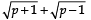is rational.
Sol:
If possible let there be a positive integer p for which= a/b is equal to a rational i.e. where a and b are positive integers.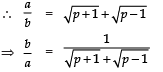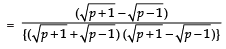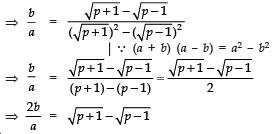Now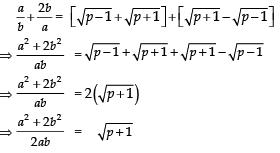Also,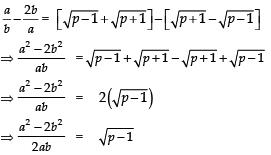Since a, b are integers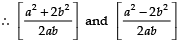are rationals

⇒ (p + 1) and (p – 1) are perfect squares of positive integers, which is not possible (because any two perfect squares differ at least by 3). Hence, there is no positive integer p for which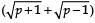is rational.

Ques 34: Prove that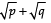is irrational, where p and q are primes.
Sol: Letbe rational
Let it be equal to ‘r’
i.e.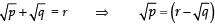Squaring both sides, we have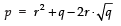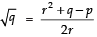...(i)
Since, p, q are both rationals
Also, r2 is rational (∵ r is rational)
∴ RHS of (i) is a rational number
⇒ LHS of (i) should be rational i.e.q  should be rational.
But q is irrational (∵ p is prime).
∴ We have arrived at a contradiction.
Thus, our supposition is wrong.
Hence, p+√q  is irrational.

Ques 35: Prove that √2+√3 is irrational.
Sol:
Let us suppose that 2+√3 is rational.
Also, let 2+√3 = a, where ‘a’ is rational
2 =  (a-√3)
Squaring both sides, we have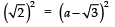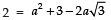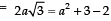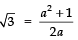i.e.√3 = a rational number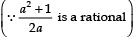But it is a contradiction (√3 is irrational)
Hence, 2+√3 is irrational.

Offer running on EduRev: Apply code STAYHOME200 to get INR 200 off on our premium plan EduRev Infinity!

132 docs

,

,

,

,

,

,

,

,

,

,

,

,

,

,

,

,

,

,

,

,

,

;